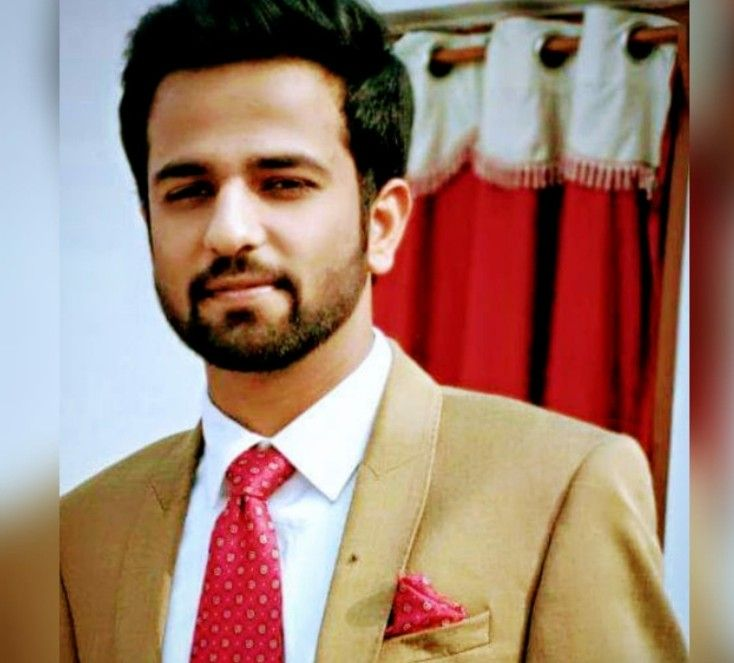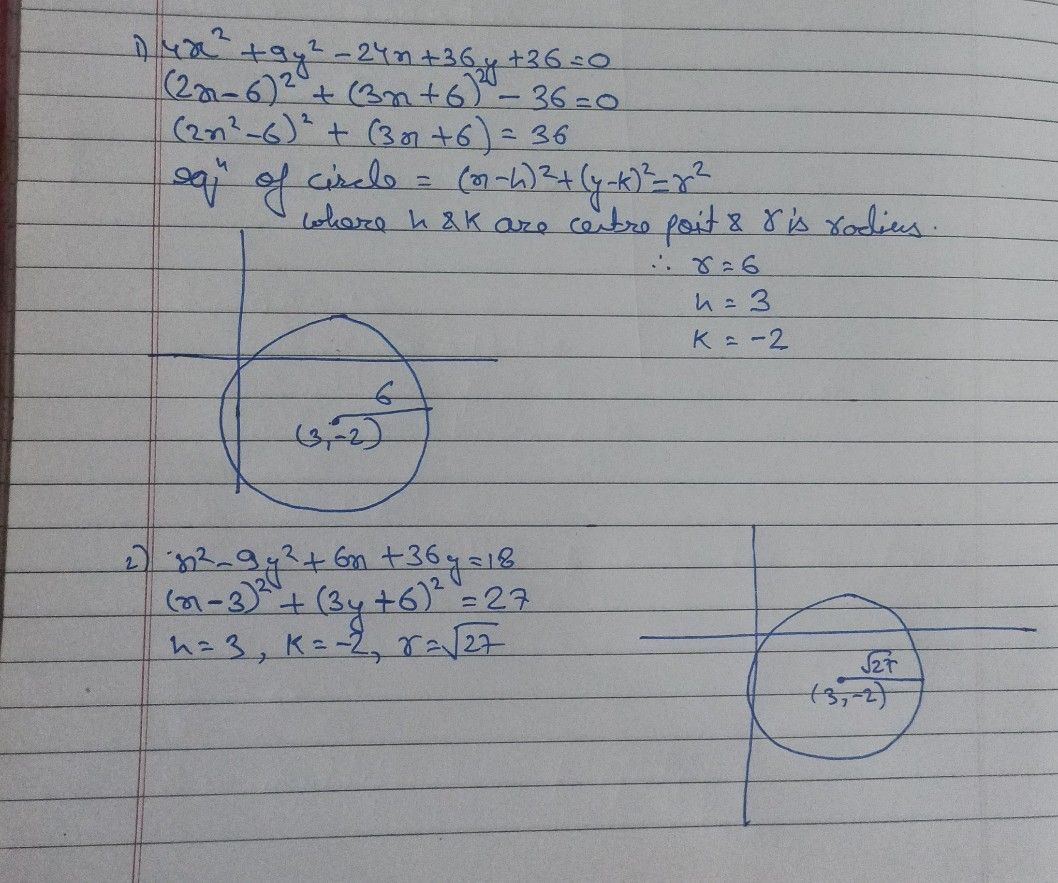Symbol
Problem$4$ B. Sketch and label the parts of the given general formula $\left(5$ (5pts each). $\right).$ – $1.$ $4x^{2}+9y^{2}-24x+36y+36=0$ – $2.$ $x^{2}-9y^{2}+6x+36y=18$